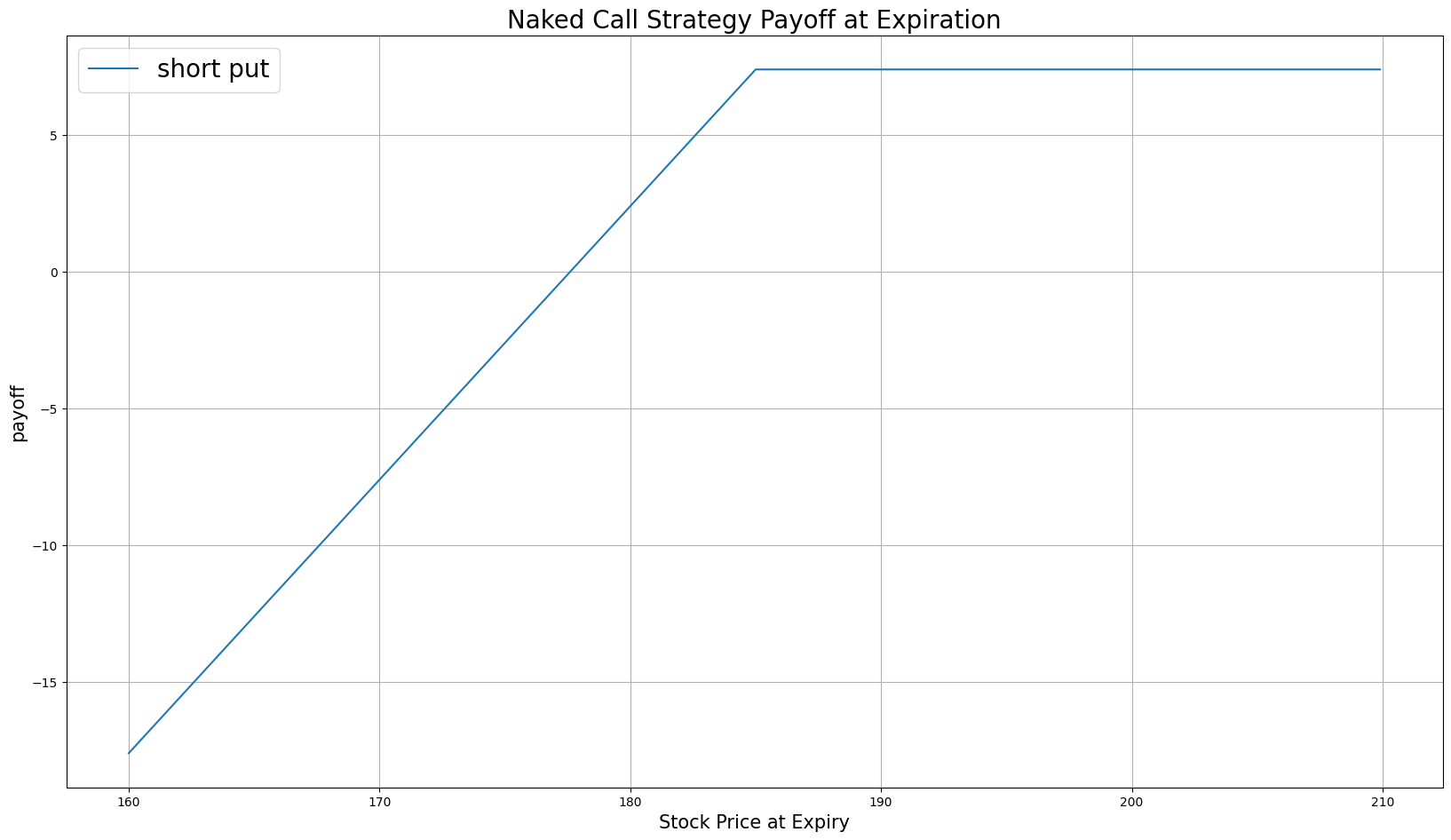# Option Strategies

## Naked Put

### Introduction

A Naked Put, also known as an uncovered put, consists of selling put Options without having a corresponding short position in the underlying security. It's called "naked" because you don't have any cover or protection in the form of a short position in the underlying asset, which exposes you to potentially unlimited risk. Naked puts aim to profit from the Option premium by selling puts. At any time for American Options or at expiration for European Options, if the stock moves above the strike price, you keep the premium. If the underlying price moves below the strike, the Option buyer can exercise the Options contract, which means you need sell the underlying at market price to fulfill your obligation to buy it at the strike price and keep the premium.

### Implementation

Follow these steps to implement the naked put strategy:

1. In the Initialize method, set the start date, end date, starting cash, and Options universe.
2. private Symbol _put, _symbol;

public override void Initialize()
{
SetStartDate(2014, 1, 1);
SetEndDate(2014, 3, 1);
SetCash(100000);

_symbol = option.Symbol;
option.SetFilter(-3, 3, 0, 31);
}
def Initialize(self) -> None:
self.SetStartDate(2014, 1, 1)
self.SetEndDate(2014, 3, 1)
self.SetCash(100000)

self.symbol = option.Symbol
self.put = None

option.SetFilter(-3, 3, 0, 31)
3. In the OnData method, select the Option contract.
4. public override void OnData(Slice slice)
{
if (_put != null && Portfolio[_put].Invested) return;

if (!slice.OptionChains.TryGetValue(_symbol, out var chain)) return;

// Find ATM put with the farthest expiry
var expiry = chain.Max(x => x.Expiry);
var atmCall = chain
.Where(x => x.Right == OptionRight.Call && x.Expiry == expiry)
.OrderBy(x => Math.Abs(x.Strike - chain.Underlying.Price))
.FirstOrDefault();
def OnData(self, slice: Slice) -> None:
if self.put and self.Portfolio[self.put].Invested:
return

chain = slice.OptionChains.get(self.symbol)
if not chain:
return

# Find ATM put with the farthest expiry
expiry = max([x.Expiry for x in chain])
put_contracts = sorted([x for x in chain
if x.Right == OptionRight.Call and x.Expiry == expiry],
key=lambda x: abs(chain.Underlying.Price - x.Strike))

if not put_contracts:
return

atm_put = put_contracts
5. In the OnData method, call the OptionStrategies.NakedPut method and then submit the order.
6. var nakedCall = OptionStrategies.NakedPut(_symbol, atmCall.Strike, expiry);

_put = atmCall.Symbol;
naked_put = OptionStrategies.NakedPut(self.symbol, atm_put.Strike, expiry)

self.put = atm_put.Symbol

Option strategies synchronously execute by default. To asynchronously execute Option strategies, set the asynchronous argument to Falsefalse. You can also provide a tag and order properties to the Buy method.

Buy(optionStrategy, quantity, asynchronous, tag, orderProperties);

self.Buy(option_strategy, quantity, asynchronous, tag, order_properties)


### Strategy Payoff

The payoff of the strategy is

$$\begin{array}{rcll} P^{K}_T & = & (K - S_T)^{+}\\ P_T & = & (P^{K}_0 - P^{K}_T)\times m - fee \end{array}$$ $$\begin{array}{rcll} \textrm{where} & P^{K}_T & = & \textrm{Put value at time T}\\ & S_T & = & \textrm{Underlying asset price at time T}\\ & K & = & \textrm{Put strike price}\\ & P_T & = & \textrm{Payout total at time T}\\ & P^{K}_0 & = & \textrm{Put price when the trade opened (credit received)}\\ & m & = & \textrm{Contract multiplier}\\ & T & = & \textrm{Time of expiration} \end{array}$$

The following chart shows the payoff at expiration:The maximum profit is $P^{K}_0$, which occurs when the underlying price is at or above the strike price of the put at expiration.

The maximum loss is $K - P^{K}_0$, which ocurrs when the underlying price drops to zero.

If the Option is American Option, there is risk of early assignment on the sold contract.

### Example

The following table shows the price details of the assets in the algorithm:

AssetPrice ($)Strike ($)
Put1.37185.00
Underlying Equity at expiration190.01-

Therefore, the payoff is

$$\begin{array}{rcll} P^{K}_T & = & (K - S_T)^{+}\\ & = & (185 - 190.01)^{+}\\ & = & 0.0\\ P_T & = & (P^{K}_0 - P^{K}_T)\times m - fee\\ & = & (1.37 - 0.0)\times m - fee\\ & = & 1.37 \times 100 - 1\\ & = & 136 \end{array}$$

So, the strategy gains \$136.

The following algorithm implements a naked put strategy:

You can also see our Videos. You can also get in touch with us via Discord.